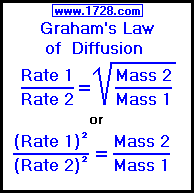Graham's Law of Diffusion Calculator Scroll to the bottom for instructions and four examples.The equation at the top of this page is Graham's Law of Diffusion, named after the Scottish chemist Thomas Graham (1805 - 1869). It states that the diffusion rate of a gas is inversely proportional to the square root of its molar mass (molecular weight). Stated in simpler terms, lighter gases (in terms of density or molecular weight) will diffuse faster than those gases with higher densities or molecular weight. Diffusion is the process in which one gas distributes itself within another, such as the way perfume is disseminated throughout a room. Effusion is the manner in which a gas escapes from a container. All containers have extremely small "holes" in them through which a gas may escape such as the way helium "escapes" from a balloon in just a few days. Graham's Law of Diffusion applies to both the diffusion and effusion rates of gases.

 Do you want to solve for:   or     or     or     ? >>>>> >>>>> >>>>> >>>>> E X A M P L E S 1) How much faster will hydrogen diffuse than a gas that has a molecular mass of 10? The atomic mass of hydrogen is 1.0079 and since hydrogen is diatomic, the molecular mass of H₂ is 2.0158 (mass 1). The other gas has a molecular mass of 10 (mass 2) and we will assign its rate the value of 1 (rate 2). We must now solve for Rate 1. Looking at Graham's Law, we can see that: (Rate 1)² = (Rate 2)² × Mass 2 ÷ Mass 1     (Rate 1)² = 1² × 10 ÷ 2.0158 Rate 1 = 2.2273 2) How much faster will hydrogen diffuse than chlorine? Both chlorine (atomic mass 35.453) and hydrogen (atomic mass 1.0079) are diatomic and so their molecular masses are 70.906 (Mass 1) and 2.0158 (Mass 2). We will assign the value of 1 (rate 1) for chlorine's rate of diffusion. We must solve for Rate 2. We can rearrange Graham's Law: (Rate 2)² = (Rate 1)² × Mass 1 ÷ Mass 2     then we plug in the numbers: (Rate 2)² = 1² × 70.906 ÷ 2.0156 Rate 2 = 5.9309 3) Oxygen diffuses 1.4886 times faster than chlorine. If we know (from problem #2) that the molecular mass of chlorine is 70.906, then what is the molecular mass of oxygen? Mass 1 = (Rate 2)² × Mass 2 ÷ (Rate 1)² Mass 1 = 1² × 70.906 ÷ 1.4886² Mass 1 = 31.998 4) What is the molecular weight of a gas that diffuses 4 times slower than hydrogen? The atomic mass of hydrogen is 1.0079 and since hydrogen is diatomic, the molecular mass of H₂ is 2.0158 (mass 1). We will give hydrogen's diffusion rate a value of 4 (rate 1) and the rate of the other gas is simply 1 (rate 2). We now have have to solve for Mass 2 and so we algebraically rearrange Graham's Law producing this formula: Mass 2 = (Rate 1)² × Mass 1 ÷ (Rate 2)²     then we plug in the numbers: Mass 2 = 4² × 2.0158 ÷ 1²     giving us our answer Mass 2 = 32.253 Significant Figures >>> The default setting is for 5 significant figures but you can change that by inputting another number in the box above. Answers are displayed in scientific notation and for easier readability, numbers between .001 and 1,000 will be displayed in standard format (with the same number of significant figures.) The answers should display properly but there are a few browsers that will show no output whatsoever. If so, enter a zero in the box above. This eliminates all formatting but it is better than seeing no output at all. Return To Home Page Copyright © 1999 - 1728 Software Systems